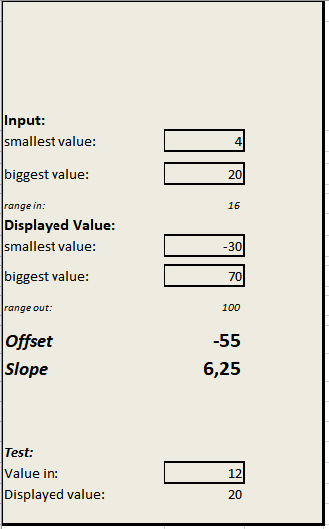# Calculating slope and offset for analog inputsCalculating slope and offset for analog inputs is much easier with this little tool:

It helps to calculate from voltage to engineer's unit in our airQlog-data logger. In the example above we calculate the slope and offset values for a temperature sensor with a range of -30°C to 70°C and a output signal of 4 to 20 mA.

I hope you find this file useful,

Andreas

Posted on Categories Howto